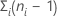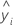# Methods and formulas for the analysis of variance in Analyze Definitive Screening Design

The adjusted sums of squares does not depend on the order that the terms enter the model. The adjusted sum of squares is the amount of variation explained by a term, given all other terms in the model, regardless of the order that the terms enter the model.

For example, if you have a model with three factors, X1, X2, and X3, the adjusted sum of squares for X2 shows how much of the remaining variation the term for X2 explains, given that the terms for X1 and X3 are also in the model.

The calculations for the adjusted sums of squares for three factors are:

• SSR(X3 | X1, X2) = SSE (X1, X2) - SSE (X1, X2, X3) or
• SSR(X3 | X1, X2) = SSR (X1, X2, X3) - SSR (X1, X2)

where SSR(X3 | X1, X2) is the adjusted sum of squares for X3, given that X1 and X2 are in the model.

• SSR(X2, X3 | X1) = SSE (X1) - SSE (X1, X2, X3) or
• SSR(X2, X3 | X1) = SSR (X1, X2, X3) - SSR (X1)

where SSR(X2, X3 | X1) is the adjusted sum of squares for X2 and X3, given that X1 is in the model.

You can extend these formulas if you have more than 3 factors in your model1.

1. J. Neter, W. Wasserman and M.H. Kutner (1985). Applied Linear Statistical Models, Second Edition. Irwin, Inc.

## Sum of squares (SS)

In matrix terms, these are the formulas for the different sums of squares:

Minitab breaks down the SS Model component into the amount of variation explained by each term or set of terms using both the sequential sum of squares and adjusted sum of squares.

### Notation

TermDescription
bvector of coefficients
Xdesign matrix
Yvector of response values
nnumber of observations
Jn by n matrix of 1s

## Sequential sum of squares

Minitab breaks down the SS Model component of variance into sequential sums of squares for each factor term or set of factor terms. The sequential sums of squares depend on the order that the factors or predictors enter the model. The sequential sum of squares is the unique portion of the SS Model that each term explains, given any terms that previously entered.

For example, if you have a model with three factors, X1, X2, and X3, the sequential sum of squares for X2 shows how much of the remaining variation X2 explains, given that X1 is already in the model. To obtain a different sequence of terms, repeat the analysis and enter the terms in a different order.

## Degrees of freedom (DF)

Different sums of squares have different degrees of freedom.

DF for a numeric factor = 1

DF for a categorical factor = b − 1

DF for a quadratic term = 1

DF for blocks = c − 1

DF for error = n − p

DF for pure error =DF for lack of fit = m − p

DF total = n − 1

For interactions among factors, multiply the degrees of freedom for the terms in the factor. For example, if the factors are A and B then the interaction AB has these degrees of freedom:
To find the degrees of freedom for a type of term, sum the degrees of freedom for the terms. For example, if the factors are A and B then the main effects in the model have this many degrees of freedom:
###### Note

Categorical factors in screening designs in Minitab have 2 levels. Thus, the degrees of freedom for a categorical factor are 2 – 1 = 1. By extension, interactions between factors also have 1 degree of freedom.

### Notation

TermDescription
bThe number of levels in the factor
cThe number of blocks
nThe total number of observations
niThe number of observations for ith factor level combination
mThe number of factor level combinations
pThe number of coefficients

### Notation

TermDescriptionThe mean of the response variableThe ith fitted value of the response
pThe number of terms in the model, not including the constant term

The calculation for the Mean Square (MS) for the model term is:

The Mean Square of the error (also abbreviated as MS Error or MSE, and denoted as s2) is the variance around the fitted regression line. The formula is:

### Notation

TermDescription
yiith observed response valueith fitted response
nnumber of observations
pnumber of coefficients in the model, not counting the constant

## F

The calculation of the F-statistic depends on the hypothesis test, as follows:

F(Term)
F(Lack-of-fit)

### Notation

TermDescription
Adj MS TermA measure of the amount of variation that a term explains after accounting for the other terms in the model.
MS ErrorA measure of the variation that the model does not explain.
MS Lack-of-fitA measure of variation in the response that could be modeled by adding more terms to the model.
MS Pure errorA measure of the variation in replicated response data.
1. J. Neter, W. Wasserman and M.H. Kutner (1985). Applied Linear Statistical Models, Second Edition. Irwin, Inc.

## P-value – Analysis of variance table

The p-value is a probability that is calculated from an F-distribution with the degrees of freedom (DF) as follows:

Numerator DF
sum of the degrees of freedom for the term or the terms in the test
Denominator DF
degrees of freedom for error

1 − P(Ffj)

### Notation

TermDescription
P(Ff)cumulative distribution function for the F-distribution
ff-statistic for the test

## P-value – Lack-of-fit test

This p-value is for the test of the null hypothesis that the coefficients are 0 for any terms that are possible to estimate from these data that are not in the model. The p-value is the probability from an F distribution with degrees of freedom (DF) as follows:
Numerator DF
degrees of freedom for lack-of-fit
Denominator DF
degrees of freedom for pure error

1 − P(Ffj)

### Notation

TermDescription
P(Ffj)cumulative distribution function for the F-distribution
fjf-statistic for the test